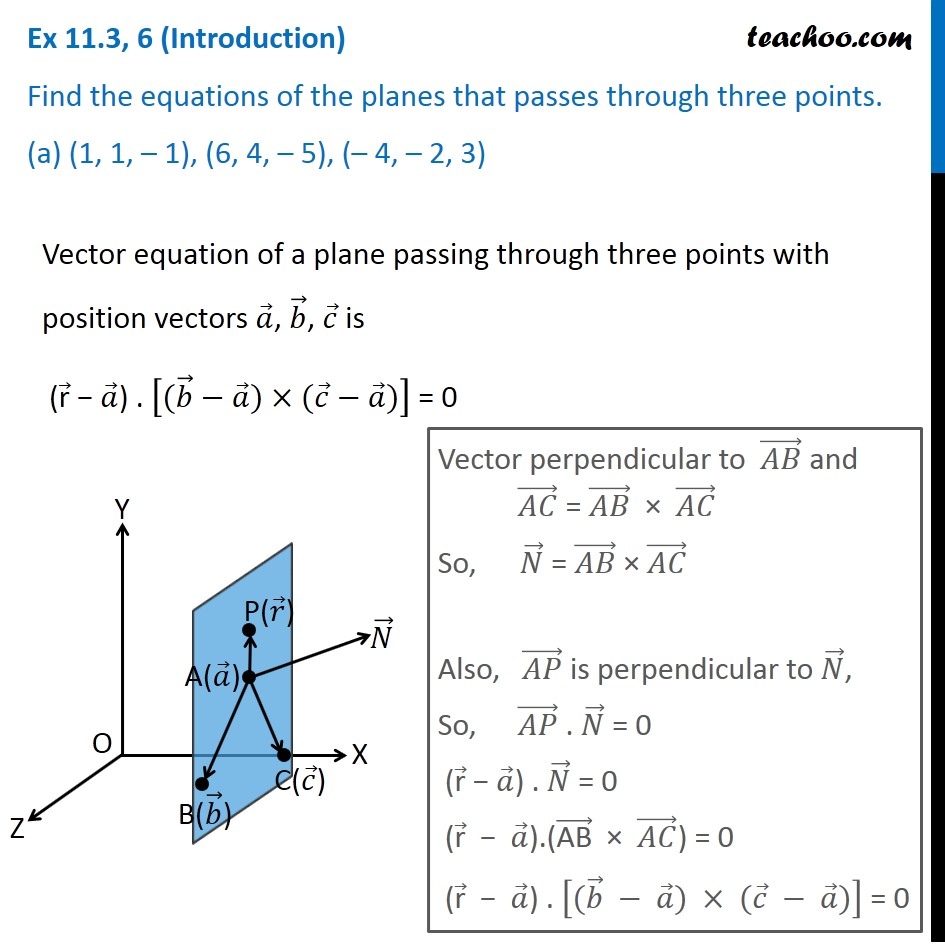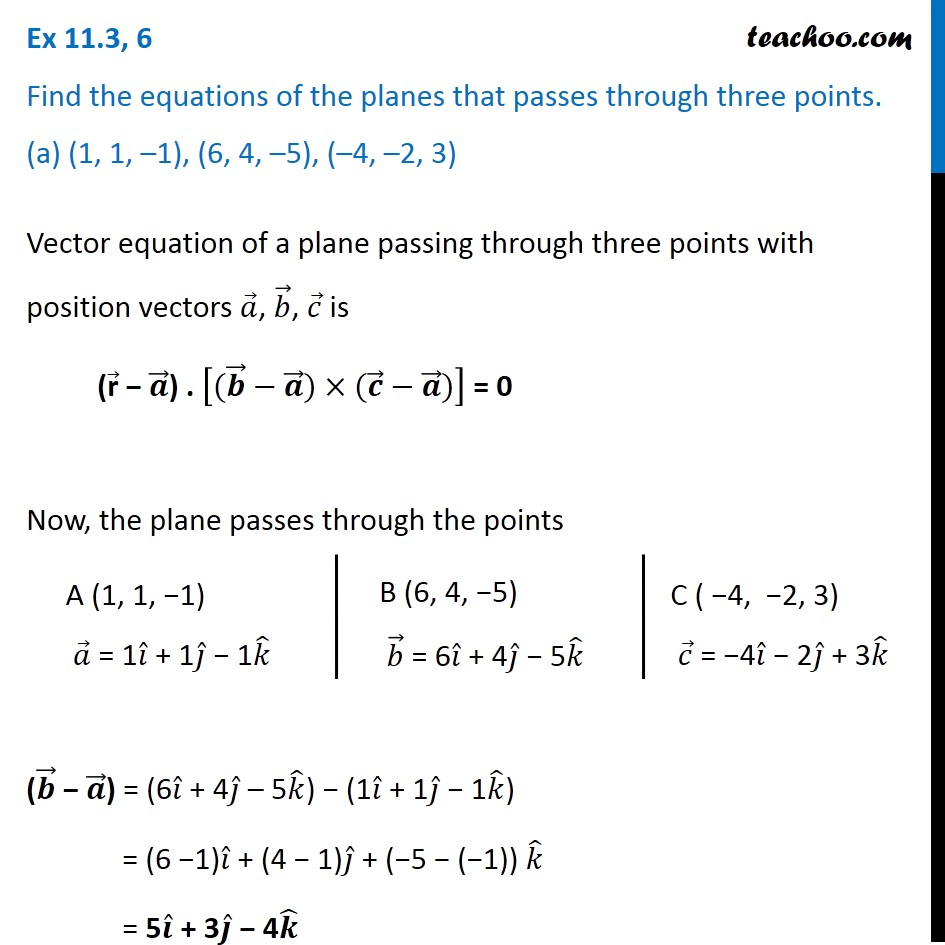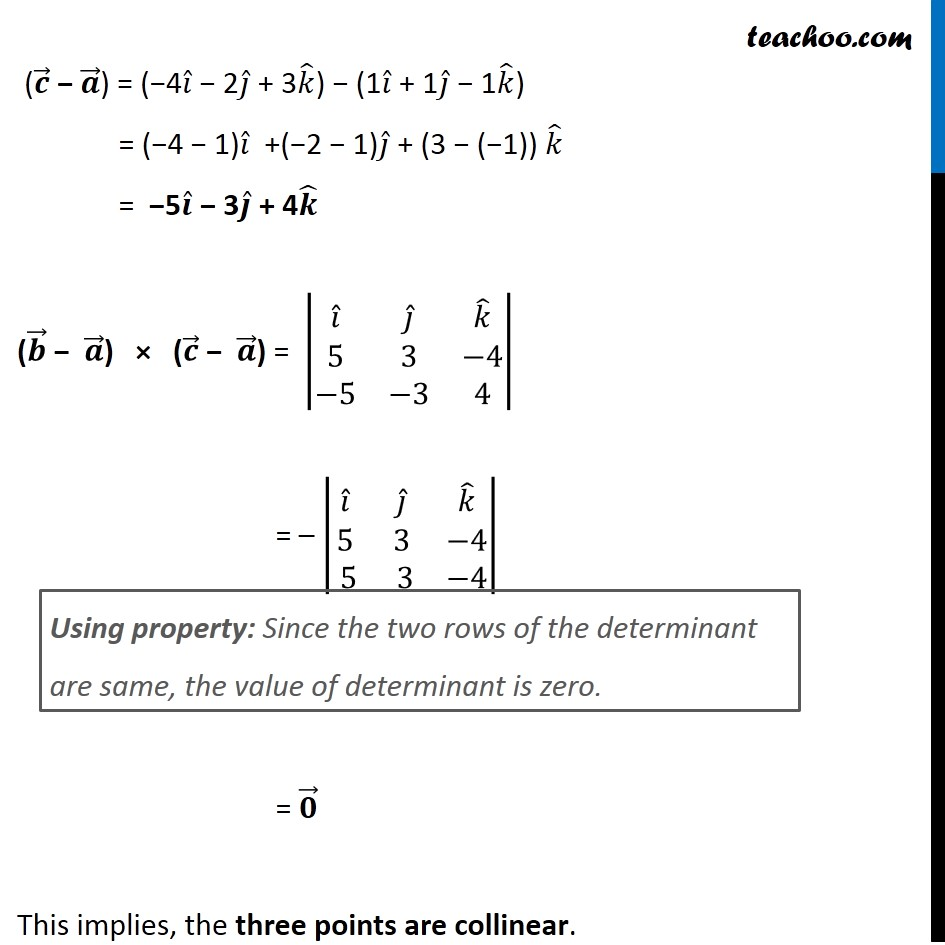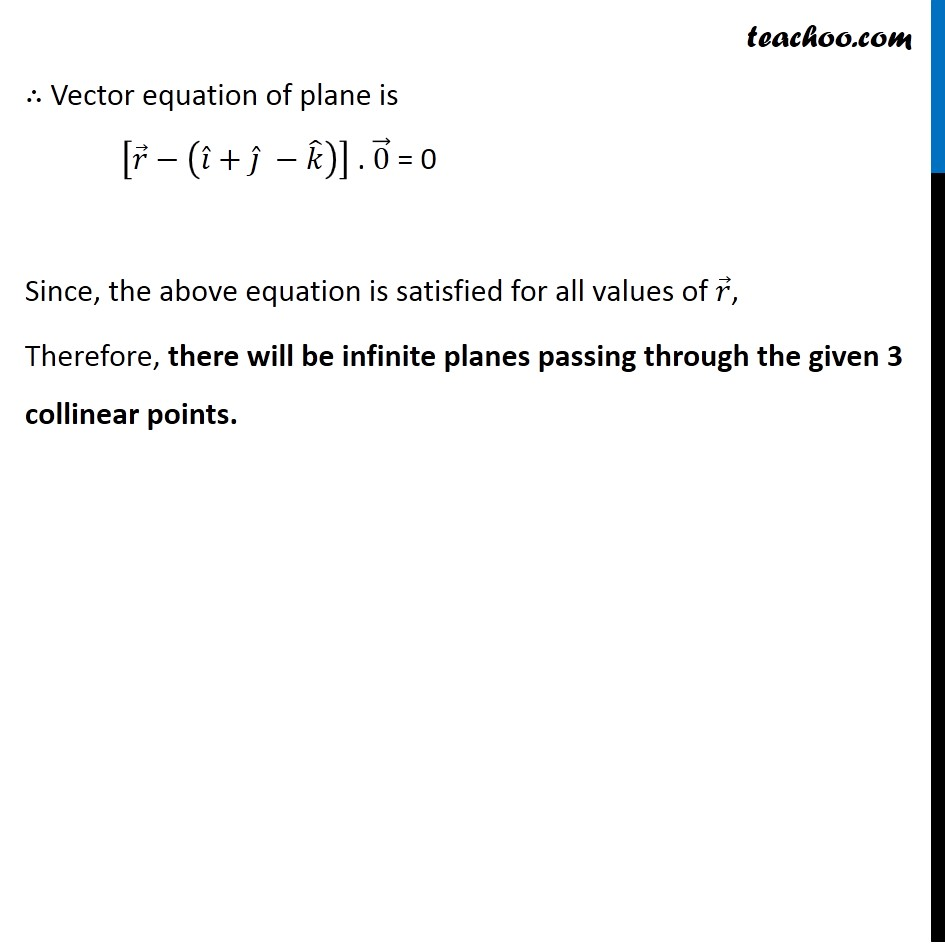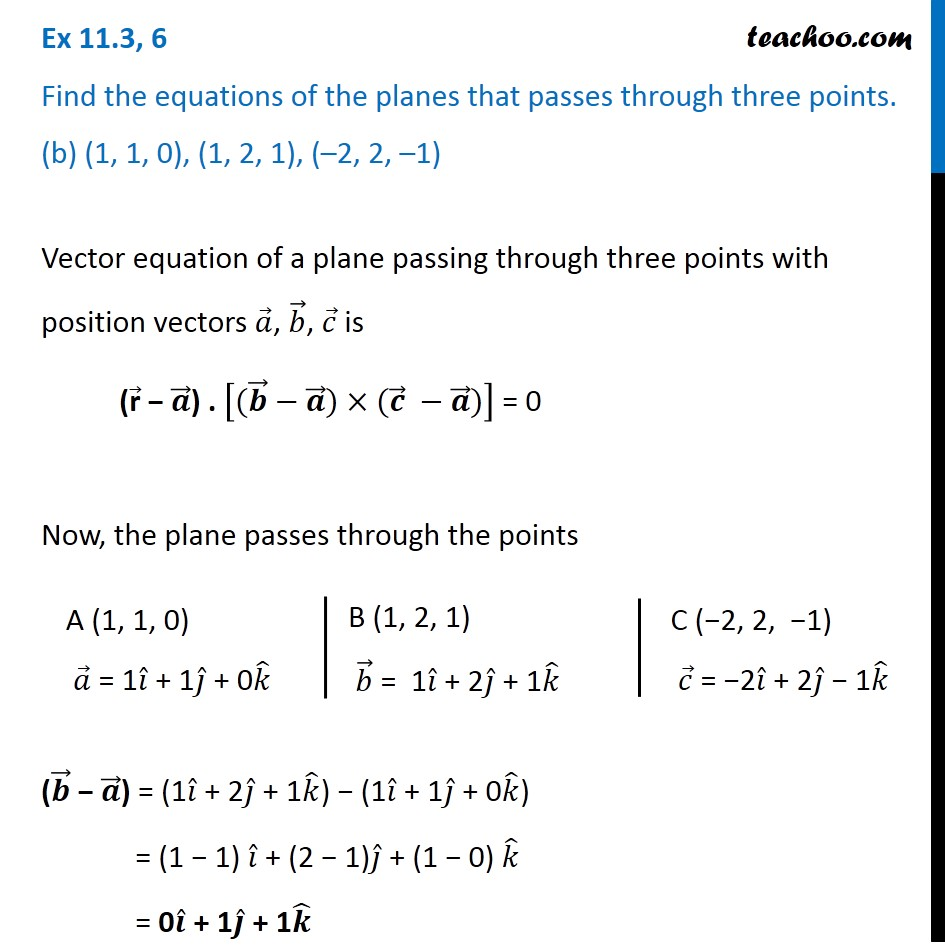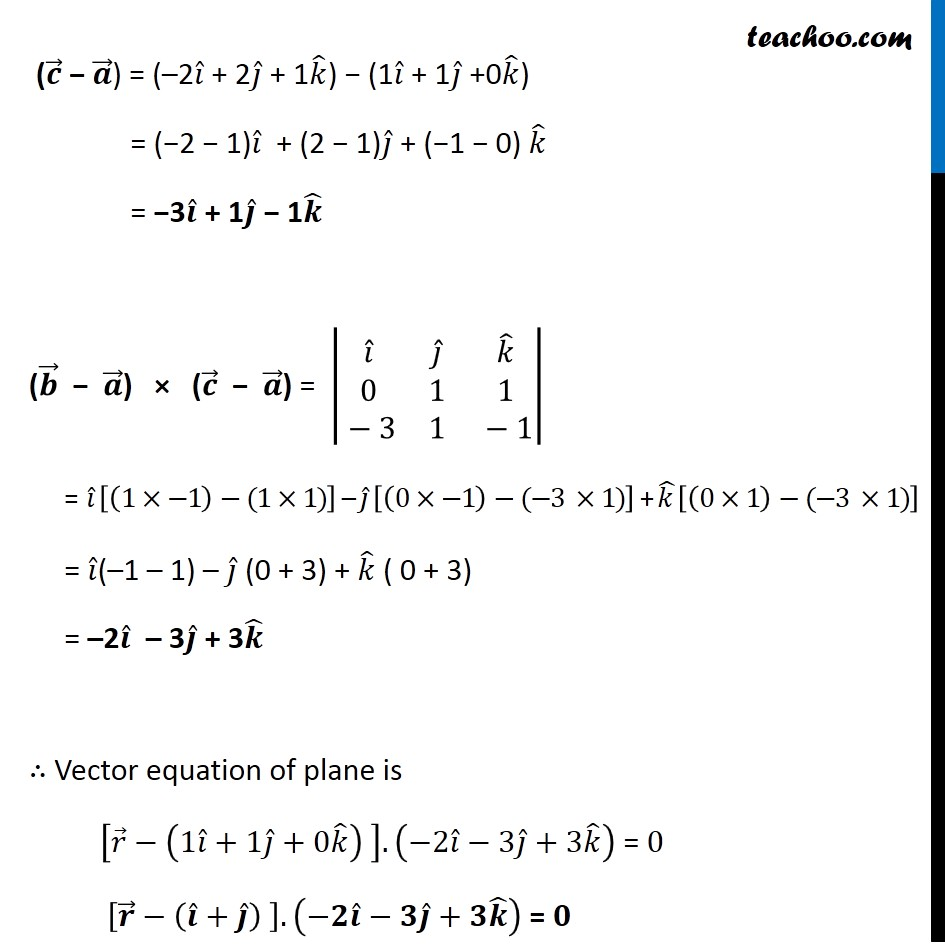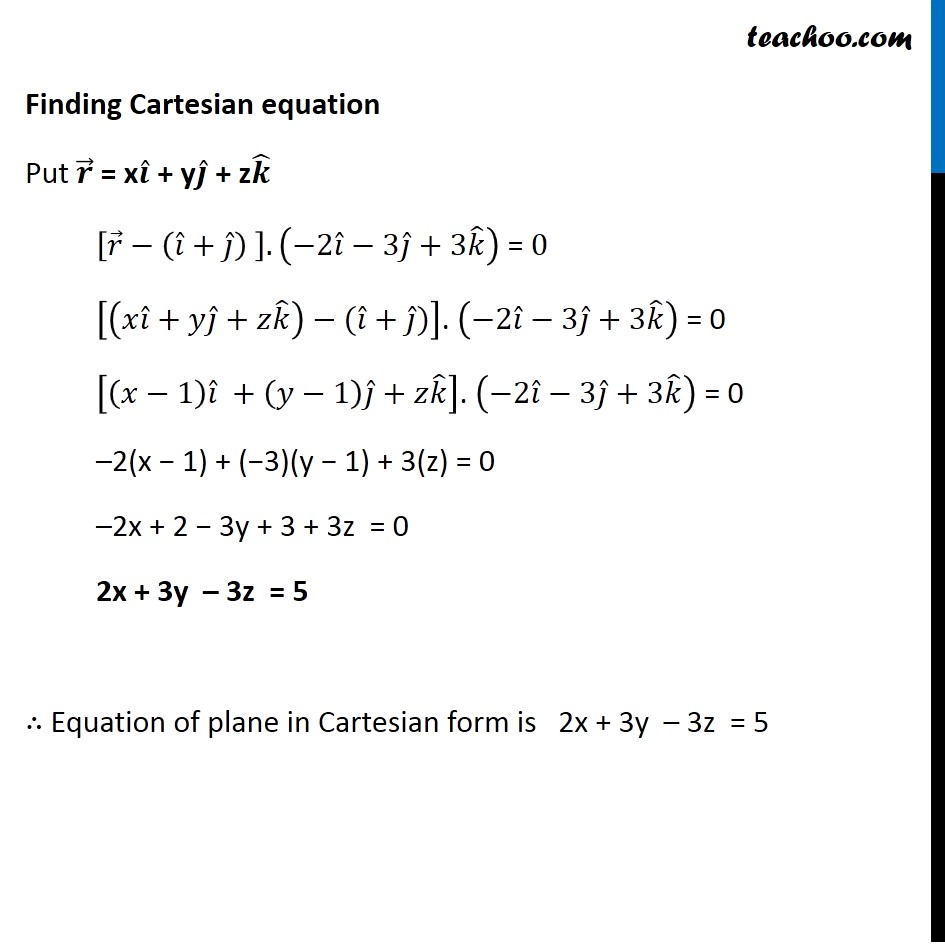Subscribe to our Youtube Channel - https://you.tube/teachoo

1. Chapter 11 Class 12 Three Dimensional Geometry
2. Serial order wise
3. Ex 11.3

Transcript

Ex 11.3, 6 (Introduction) Find the equations of the planes that passes through three points. (a) (1, 1, – 1), (6, 4, – 5), (– 4, – 2, 3) Vector equation of a plane passing through three points with position vectors 𝑎 ⃗, 𝑏 ⃗, 𝑐 ⃗ is ("r" ⃗ − 𝑎 ⃗) . [(𝑏 ⃗−𝑎 ⃗)×(𝑐 ⃗−𝑎 ⃗)] = 0 Ex 11.3, 6 Find the equations of the planes that passes through three points. (a) (1, 1, –1), (6, 4, –5), (–4, –2, 3) Vector equation of a plane passing through three points with position vectors 𝑎 ⃗, 𝑏 ⃗, 𝑐 ⃗ is ("r" ⃗ − 𝒂 ⃗) . [(𝒃 ⃗−𝒂 ⃗)×(𝒄 ⃗−𝒂 ⃗)] = 0 Now, the plane passes through the points (𝒃 ⃗ − 𝒂 ⃗) = (6𝑖 ̂ + 4𝑗 ̂ – 5𝑘 ̂) − (1𝑖 ̂ + 1𝑗 ̂ − 1𝑘 ̂) = (6 −1)𝑖 ̂ + (4 − 1)𝑗 ̂ + (−5 − (−1)) 𝑘 ̂ = 5𝒊 ̂ + 3𝒋 ̂ − 4𝒌 ̂ A (1, 1, −1) 𝑎 ⃗ = 1𝑖 ̂ + 1𝑗 ̂ − 1𝑘 ̂ B (6, 4, −5) 𝑏 ⃗ = 6𝑖 ̂ + 4𝑗 ̂ − 5𝑘 ̂ C ( −4, −2, 3) 𝑐 ⃗ = −4𝑖 ̂ − 2𝑗 ̂ + 3𝑘 ̂ (𝒄 ⃗ − 𝒂 ⃗) = (−4𝑖 ̂ − 2𝑗 ̂ + 3𝑘 ̂) − (1𝑖 ̂ + 1𝑗 ̂ − 1𝑘 ̂) = (−4 − 1)𝑖 ̂ +(−2 − 1)𝑗 ̂ + (3 − (−1)) 𝑘 ̂ = −5𝒊 ̂ − 3𝒋 ̂ + 4𝒌 ̂ (𝒃 ⃗ − 𝒂 ⃗) × (𝒄 ⃗ − 𝒂 ⃗) = |■8(𝑖 ̂&𝑗 ̂&𝑘 ̂@5&3&−4@−5&−3&4)| = – |■8(𝑖 ̂&𝑗 ̂&𝑘 ̂@5&3&−4@ 5& 3&−4)| = 𝟎 ⃗ This implies, the three points are collinear. Using property: Since the two rows of the determinant are same, the value of determinant is zero. ∴ Vector equation of plane is [𝑟 ⃗−(𝑖 ̂+𝑗 ̂ −𝑘 ̂ )] . 0 ⃗ = 0 Since, the above equation is satisfied for all values of 𝑟 ⃗, Therefore, there will be infinite planes passing through the given 3 collinear points. Ex 11.3, 6 Find the equations of the planes that passes through three points. (b) (1, 1, 0), (1, 2, 1), (–2, 2, –1) Vector equation of a plane passing through three points with position vectors 𝑎 ⃗, 𝑏 ⃗, 𝑐 ⃗ is ("r" ⃗ − 𝒂 ⃗) . [(𝒃 ⃗−𝒂 ⃗)×(𝒄 ⃗ −𝒂 ⃗)] = 0 Now, the plane passes through the points A (1, 1, 0) 𝑎 ⃗ = 1𝑖 ̂ + 1𝑗 ̂ + 0𝑘 ̂ B (1, 2, 1) 𝑏 ⃗ = 1𝑖 ̂ + 2𝑗 ̂ + 1𝑘 ̂ C (−2, 2, −1) 𝑐 ⃗ = −2𝑖 ̂ + 2𝑗 ̂ − 1𝑘 ̂ (𝒃 ⃗ − 𝒂 ⃗) = (1𝑖 ̂ + 2𝑗 ̂ + 1𝑘 ̂) − (1𝑖 ̂ + 1𝑗 ̂ + 0𝑘 ̂) = (1 − 1) 𝑖 ̂ + (2 − 1)𝑗 ̂ + (1 − 0) 𝑘 ̂ = 0𝒊 ̂ + 1𝒋 ̂ + 1𝒌 ̂ (𝒄 ⃗ − 𝒂 ⃗) = (–2𝑖 ̂ + 2𝑗 ̂ + 1𝑘 ̂) − (1𝑖 ̂ + 1𝑗 ̂ +0𝑘 ̂) = (−2 − 1)𝑖 ̂ + (2 − 1)𝑗 ̂ + (−1 − 0) 𝑘 ̂ = −3𝒊 ̂ + 1𝒋 ̂ − 1𝒌 ̂ (𝒃 ⃗ − 𝒂 ⃗) × (𝒄 ⃗ − 𝒂 ⃗) = |■8(𝑖 ̂&𝑗 ̂&𝑘 ̂@0&1&1@ − 3&1& − 1)| = 𝑖 ̂ [(1×−1)−(1×1)] – 𝑗 ̂ [(0×−1)−(−3 ×1)] + (𝑘 ) ̂[(0×1)−(−3 ×1)] = 𝑖 ̂(–1 – 1) – 𝑗 ̂ (0 + 3) + 𝑘 ̂ ( 0 + 3) = –2𝒊 ̂ – 3𝒋 ̂ + 3𝒌 ̂ ∴ Vector equation of plane is [𝑟 ⃗−(1𝑖 ̂+1𝑗 ̂+0𝑘 ̂ ) ].(−2𝑖 ̂−3𝑗 ̂+3𝑘 ̂ ) = 0 [𝒓 ⃗−(𝒊 ̂+𝒋 ̂ ) ].(−𝟐𝒊 ̂−𝟑𝒋 ̂+𝟑𝒌 ̂ ) = 𝟎 Finding Cartesian equation Put 𝒓 ⃗ = x𝒊 ̂ + y𝒋 ̂ + z𝒌 ̂ [𝑟 ⃗−(𝑖 ̂+𝑗 ̂ ) ].(−2𝑖 ̂−3𝑗 ̂+3𝑘 ̂ ) = 0 [(𝑥𝑖 ̂+𝑦𝑗 ̂+𝑧𝑘 ̂ )−(𝑖 ̂+𝑗 ̂)]. (−2𝑖 ̂−3𝑗 ̂+3𝑘 ̂ ) = 0 [(𝑥−1) 𝑖 ̂ +(𝑦−1) 𝑗 ̂+𝑧𝑘 ̂ ]. (−2𝑖 ̂−3𝑗 ̂+3𝑘 ̂ ) = 0 –2(x − 1) + (−3)(y − 1) + 3(z) = 0 –2x + 2 − 3y + 3 + 3z = 0 2x + 3y – 3z = 5 ∴ Equation of plane in Cartesian form is 2x + 3y – 3z = 5

Ex 11.3